Fibonacci Sequence and Phi (Φ)The Fibonacci sequence was originally discovered by the Italian mathematician Leonardo de Fibonacci de Pisa (1170–1240). The basic concept of the Fibonacci sequence is that each number equals the sum of the two previous numbers.

The Fibonacci Sequence of Numbers Explained

As it was mentioned, Fibonacci discovered a unique numerical sequence according to which each number equals the sum of the previous two numbers, as follows:

• 1+1=2

• 1+2=3

• 2+3=5

• 3+5=8

• 5+8=13

• 8+13=21

• 13+21=34

to infinity

or else:

F(n) = F(n-2) + F(n-1)

Table: The first 60 Fibonacci Numbers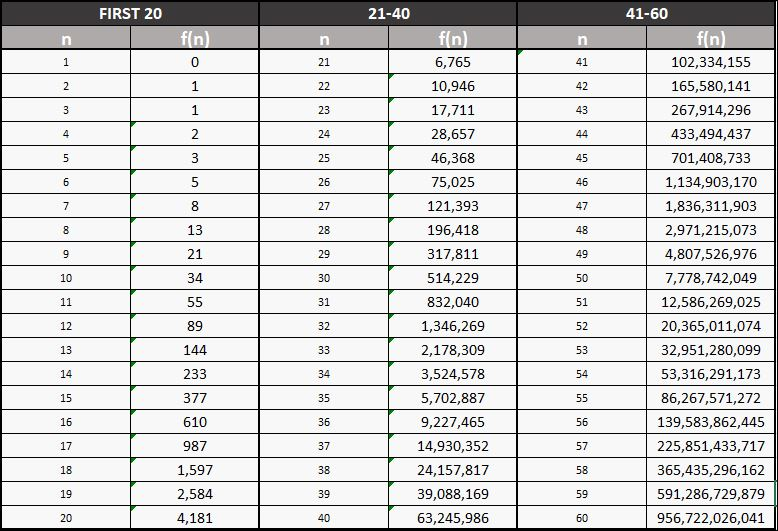The Fibonacci Ratios

If you start dividing the Fibonacci numbers the result is always close to three ratios: 1.618, 0.618, or 0.382. For example, let's take three Fibonacci numbers 144, 233, and 377:

• If you divide 233 by 144, the result is 1.618

• If you divide 144 by 233, the result is 0.618

• If you divide 144 by 377, the result is 0.382

The ratios 1.618, 0.618, and 0.382 are very important when trading stocks, Forex, or any other financial asset. The Fibonacci ratios create an extended series of trading tools that are available in all modern trading platforms.

Additionally, the Fibonacci sequence of numbers provides the background of various methodologies when trading the global markets. There are numerous theories and methods explaining the behavior of financial markets based solely on the Fibonacci numbers and their derived ratios. This sequence of numbers and their ratios can be found behind hundreds of trading systems worldwide.

Fibonacci Sequence of Numbers and Phi

As it was mentioned before, if we divide each Fibonacci number to the previous Fibonacci number the result is always close to 1.618. The number 1.618 is known as Phi (Φ).

Phi (Φ) = 1.618033… (until infinity)

The Ancient Origins of Phi

Originally, the Phi number was properly defined by the ancient mathematician Euclid (around 300-B.C.). Before Euclid, the ancient philosopher Plato (428-B.C. – 347-B.C.) considered the Golden Ratio to be the most universally binding of all mathematical relationships. Before Plato, the great mathematician Pythagoras (570-B.C. – 475-B.C.) also referred to the Golden Ratio. Actually, no one knows for sure who really discovered Phi. Some believe that ancient Egyptians initially discovered and applied it to the Pyramids, others believe that the ancient Sumerians discovered it.

Phi ‘Φ’ is the first letter of the name Phidias (Greek:Φειδίας), the ancient sculptor who created the statue of Zeus at Olympia, one of the seven wonders of the ancient world. He applied the golden ratio in the construction of the Athenian Parthenon.

Unique mathematical properties of Phi

It is impossible to pin the golden ratio down. The first 10 million digits were computed in 1996, but they were never repeated. Here are some basic mathematical properties of Phi.

Key Properties of Phi:

(i) If you square Phi (Phi²), you get the number Phi+1, or else 2.618…, and that means:

Φ² = Φ + 1 = 2.618

(ii) If you divide Phi into 1 (1/Phi) you get the number Phi-1, or else 0.618…, and that means:

1 / Φ = Φ – 1 = 0.618

0.618 is sometimes symbolized as a small ‘p’

Golden Spiral

A golden spiral in Geometry is a logarithmic spiral whose growth factor is Phi (Φ). Α golden spiral gets wider by a factor of Phi for every quarter turn it makes.

Image: Fibonacci Golden Spiral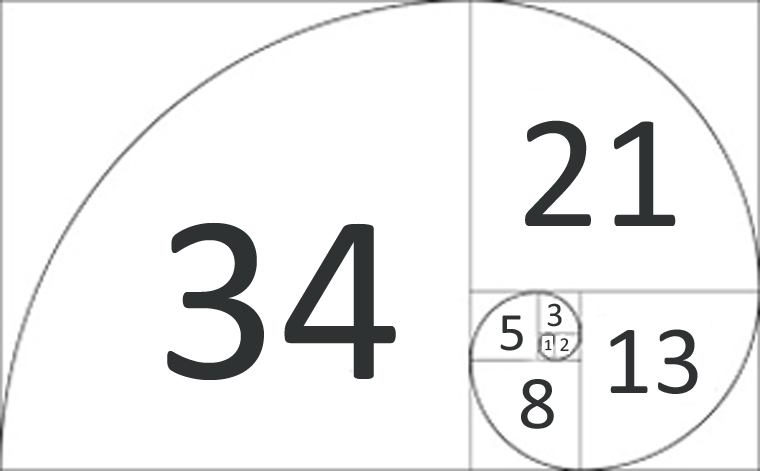More about the Golden Spiral: https://math.temple.edu/~reich/Fib/fibo.html

Applications of Phi in the Universe

Phi is found everywhere in our body, nature, and even in our universe:

• The diameters of Moon and Earth form a mathematical triangle with dimensions close to phi properties
• The distances between our solar system’s sun and its planets correlate closely to Phi exponential powers
• NASA, in 2003, released that the shape of our Universe (Milky Way) is a dodecahedron based on Phi. The Milky Way has a number of spiral arms, each of which has a logarithmic spiral of roughly 12 degrees. The shape of the spiral is identical to the Golden Spiral.

Image: Applying two Golden Spirals on the nearby spiral galaxy M74 (Qexpert.com, original image: NASA/ESA Hubble Space Telescope)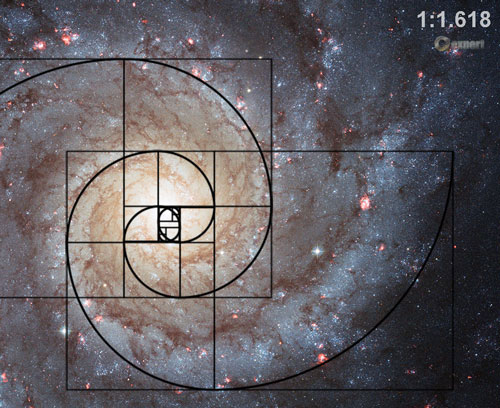Fibonacci Sequence and Phi (Φ)

Trading Tutorials: » Introduction to Money Management | » Essential Forex Trading Tips |### Online Forex BrokersFibonacci mathematics aims to reveal the hidden proportionality of market behavior. Find Fibonacci trading tools and tutorials:

eBOOKTRADING WORLD MARKETS USING PHI AND THE FIBONACCI NUMBERS (2018)

Complete Guide to Fibonacci Trading with Reference to Elliott Waves, Gann Numbers, and Harmonic Patterns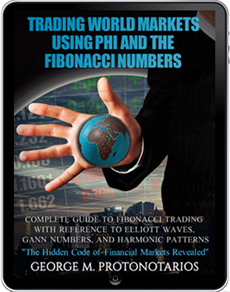### 2022 Holidays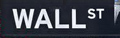Date Holiday Jan. 17, Monday Martin Luther King Feb. 21, Monday Presidents' Day April 15, Friday Good Friday May 30, Monday Memorial Day June 20, Monday Juneteenth Day July 4, Monday Independence Day Sept. 5, Monday Labor Day Nov. 24, Thursday Thanksgiving Day Dec. 26, Monday Christmas Day

### Chart PatternsIdentify and Trade Reliable Chart Patterns: